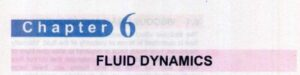Hello, students in this post we will discuss Fsc 1st Year Physics Chapter 6 Solved Exercise. In previous post we discuss the chapter 5 solution now discuss the chapter 6 exercise short question in a simple way that will helps you to get high marks in exams. So let get started.

## Fsc 1st Year Physics Chapter 6 Solved Exercise

Q 6.1: Solution

• The frictional effect existing between the layers of fluids is called viscosity. Viscosity finds the value of force needed to move one layer of liquid over the other. Its system international unit is Newton second per meter square. η used for its representation

Q 6.2: Solution

• When object moves in the fluids bear the retarding force known as a drag force. If the speed of an object in the fluids increases it faces larger drag force
• For spherical objects equation for drag force is
• F=6Πηrv
• r denotes the radius of the spherical body
• v is the speed of the body
• η coefficient of viscosty

Q 6.3: Solution

• The equation for terminal velocity is
• Vt=mg/6Πηr
• Here  g/6Πηr is constant
• hence
• Vt is directly proportional to m
• As mass of the droplet is small so terminal velocity is small. so it looks suspended in the air

Q 6.4: Solution

• In laminar flow, if every particle of liquid follows the path that its earlier fluid particle has followed.
• The irregular flow of liquid is called turbulent flow. In this flow path is constantly changing and high level of discordance is exist

Q 6.5: Solution

Q 6.6: Solution

• On the basis of Bernoulli’s equation pressure is low where the speed of fluid is high so when man standing near the fast moving train the speed of air between man and train is high and pressure is low and behind the man pressure is high and speed of air is low. So man get the force from higher to low pressure.

Q 6.7: Solution

• 1 is correct where the speed of fluid is high pressure is low

Q 6.8: Solution

• On the basis of Bernoulli’s equation, we know that pressure will be low where speed is high and vice versa
• So when to boats move with high speed in parallel to each other in same direction. The speed of water between two boats is high than other sides so pressure exerts on them from the low-speed side and comes close to each other.

Q 6.9: Solution

• Pressure will be low where speed is high and vice versa on the basis of the Bernoulli equation.
• So when a cricket ball is through the speed of air on the shining side will be high and pressure is low and on the rough side pressure is high speed is low. In result, ball moves form high pressure to low pressure in the curve path and it is a swing of ball.

Q 6.10: Solution

• In the carburetor of car there is a venturi duct used to feed the accurate mixture of air and petrol to the cylinder Air is drawn through the duct along the cylinder’s pipe. There is a small inlet at the side of duct fed with petrol
• Air moves in the duct with a high speed and makes low pressure that converts the petrol into vapor can seen here
• On the level of heart blood pressure is measured
• When horizontally lying the blood not have to do high work against gravity since all parts of the body in level with the heart.
• Hence c is the correct answer

Q 6.12: Solution

• On in the case of weightlessness blood pressure will be the same in major parties of leg and neck in orbiting space station.

So students we have discussed Fsc 1st Year Physics Chapter 6 Solved Exercise. If you have any problems let me know in the comments box. Thanks for reading have a nice day